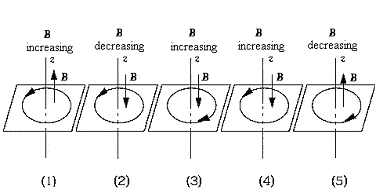# Lenz's Law and Current's Direction

## Homework Statement

A loop rests in the xy plane. The z axis is normal to the plane and positive upward. The direction of the changing flux is indicated by the arrow on the z axis. The diagram that correctly shows the direction of the resultant induced current in the loop isa. (1)
b. (2)
c. (3)
d. (4)
e. (5)

## Homework Equations

emf = - [d(magnetic flux)]/dt

## The Attempt at a Solution

In Figure 4, the magnetic flux is increasing in the -z-direction. From Lenz's Law, there is an opposition to this change, so the induced current will be counter-clockwise, which corresponds to the +z-direction?

Thanks.